SPSS TUTORIALS BASICS ANOVA REGRESSION FACTOR CORRELATION

# SPSS Cochran Q-Test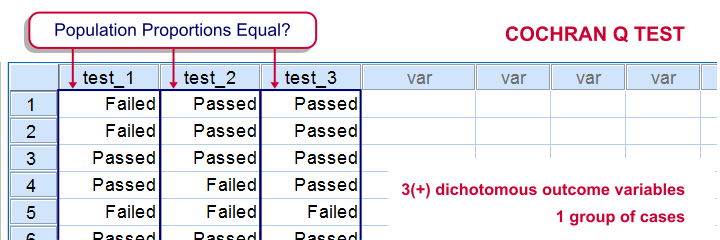SPSS Cochran Q test is a procedure for testing if the proportions of 3 or more dichotomous variables are equal in some population. These outcome variables have been measured on the same people or other statistical units.

## SPSS Cochran Q Test Example

The principal of some university wants to know whether three examns are equally difficult. Fifteen students took these examns and their results are in examn_results.sav.

## 1. Quick Data Check

It's always a good idea to take a quick look at what the data look like before proceeding to any statistical tests. We'll open the data and inspect some histograms by running FREQUENCIES with the syntax below. Note the TO keyword in step 3.

*1. Set default directory.

*2. Open data.

get file 'examn_results.sav'.

*3. Quick check.

frequencies test_1 to test_3
/format notable
/histogram.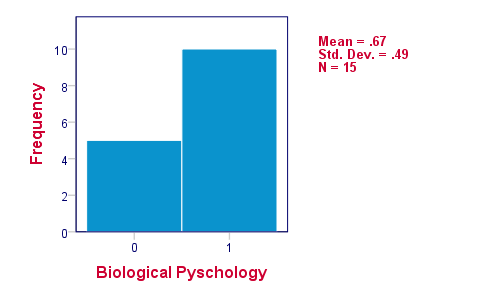The histograms indicate that the three variables are indeed dichotomous (there could have been some “Unknown” answer category but it doesn't occur). Since N = 15 for all variables, we conclude there's no missing values. Values 0 and 1 represent “Failed” and “Passed”.We suggest you RECODE your values if this is not the case. We therefore readily see that the proportions of students succeeding range from .53 to .87.

## 2. Assumptions Cochran Q Test

Cochran's Q test requires only one assumption:

• independent observations (or, more precisely, independent and identically distributed variables);

## 3. Running SPSS Cochran Q Test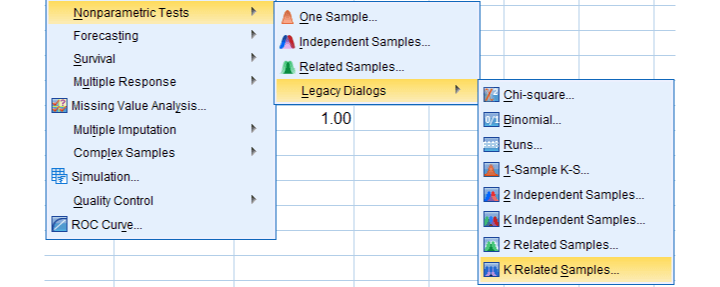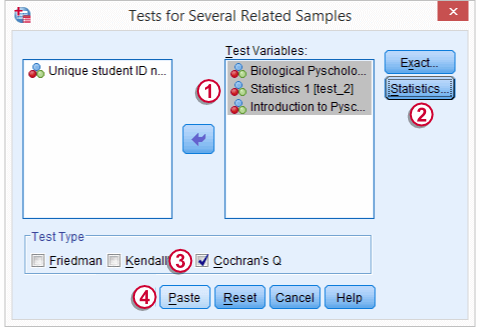We'll navigate to AnalyzeNonparametric TestsLegacy DialogsK Related Samples...We move our test variables under Test Variables,select Descriptive under Statistics,select Cochran's Q under Test Type andclick
This results in the syntax below which we then run in order to obtain our results.

*Run Cochran Q test.

NPAR TESTS
/COCHRAN=test_1 test_2 test_3
/STATISTICS DESCRIPTIVES
/MISSING LISTWISE.

## 4. SPSS Cochran Q Test Output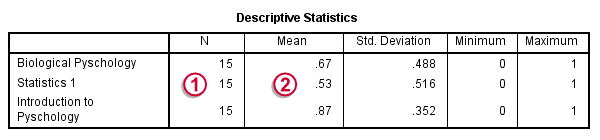The first table (Descriptive Statistics) presents the descriptives we'll report. Do not report the results from `DESCRIPTIVES` instead.The reason is that the significance test is (necessarily) based on cases without missing values on any of the test variables. The descriptives obtained from Cochran's test are therefore limited to such complete cases too.Since N = 15, the descriptives once again confirm that there are no missing values andthe proportions range from .53 to .87.Again, proportions correspond to means if 0 and 1 are used as values.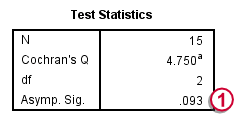The table Test Statistics presents the result of the significance test.The p-value (“Asymp. Sig.”) is .093; if the three tests really are equally difficult in the population, there's still a 9.3% chance of finding the differences we observed in this sample. Since this chance is larger than 5%, we do not reject the null hypothesis that the tests are equally difficult.

## 5. Reporting Cochran's Q Test Results

When reporting the results from Cochran's Q test, we first present the aforementioned descriptive statistics. Cochran's Q statistic follows a chi-square distribution so we'll report something like “Cochran's Q test did not indicate any differences among the three proportions, χ2(2) = 4.75, p = .093”.

# Tell us what you think!

*Required field. Your comment will show up after approval from a moderator.

# THIS TUTORIAL HAS 6 COMMENTS:

•### By Ruben Geert van den Berg on July 27th, 2016

Hi Yowie!

Sorry for my late response but I finally had the time to look into this. The difference occurs because -by default- the McNemar test reports an exact p-value and the Cochran's Q test reports and approximate ("asymptotic") p-value. So if you request the exact p-value for Cochran's Q test, the outcomes are identical.

I ran all tests on your data and the syntax is accessible at McNemar Test Versus Cochran Q Test. I added the conclusions at the end. Hope you find it helpful.